# TypeScript Loops Tutorial with Examples

#### View more Tutorials: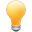Follow us on our fanpages to receive notifications every time there are new articles.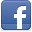Facebook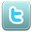Twitter

## 1- What is loop?

In TypeScript, code is executed sequentially from top to bottom. However, if you want to execute a block of code more than once, let's use a loop.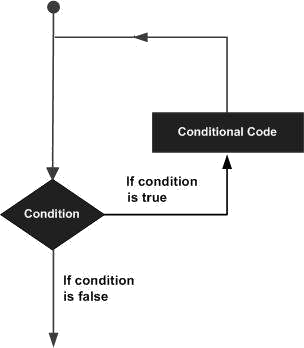TypeScript provides the following types of loops:
1. while
2. for
3. for..of
4. for..in
5. do..while
The following statements can also appear inside the loop:
• break
• continue
break
When the break statement is encountered, the program will terminate the loop immediately.
continue
When the continue statement is encountered, the program will skip the lines below the continue and execute the next iteration (If the loop condition is still true).

## 2- while loop

while loop syntax:
``````
while (condition)  {
// Do something here..
}
``````
Example:
loop_while_ex1.ts
``````
console.log("While loop example");
let x = 2;
while (x < 10) {
console.log("Value of x = ", x);
x = x + 3;
}
console.log("Done!");
``````
Output:
``````
While loop example
Value of x =  2
Value of x =  5
Value of x =  8
Done!
``````

## 3- for loop

Syntax of for loop:
``````
for (initialValues; condition; updateValues) {
// Statements to be executed repeatedly
}
``````
• InitialValues: Initialize values for related variables in the loop.
• condition: Condition to execute the block.
• updateValues: Update new values for variables.
Example:
loop_for_ex1.ts
``````
console.log("For loop example");
for (let i = 0; i < 10; i = i + 3) {
console.log("i= " + i);
}
``````
Output:
``````
For loop example
i= 0
i= 3
i= 6
i= 9
``````
Example: Two variables participate in the condition of a for loop:
loop_for_ex2.ts
``````
console.log("For loop example");
for (let i = 0, j = 0; i + j < 10; i = i + 1, j = j + 2) {
console.log("i = " + i + ", j = " + j);
}
``````
Output:
``````
For loop example
i = 0, j = 0
i = 1, j = 2
i = 2, j = 4
i = 3, j = 6
``````
Using a for loop can help you iterate over the elements of an array.
loop_for_ex3.ts
``````
console.log("For loop example");
// Array
let names =["Tom","Jerry", "Donald"];

for (let i = 0; i < names.length; i++ ) {
console.log("Name = ", names[i]);
}
console.log("Done!");
``````
Output:
``````
For loop example
Name =  Tom
Name =  Jerry
Name =  Donald
Done!
``````

## 4- for..in loop

The for..in loop is used to:
• Iterate over field names or property names of an object.
• Iterate over the indices of an array, list, or tuple.
Syntax:
``````
for (propName in object) {
// Do something
}

for (index in array) {  // array, tuple, list
// Do something
}
``````
Example: Use for..in loop to access fields and properties of an object.
loop_for_in_object_ex1.ts
``````
// An object has 4 properties (name, age, gender,greeing)
var myObject = {
name: "Tom",
age: 25,
gender: "Male",
greeting: function () {
return "Hello";
}
};
for (let myProp in myObject) {
console.log(`prop: \${myProp}`);
let key = myProp as keyof typeof myObject; // Define a key of an Object
let value = myObject[key];
console.log(`value: \${value}`);
console.log(' --------- ');
}
``````
Output:
``````
prop: name
value: Tom
---------
prop: age
value: 25
---------
prop: gender
value: Male
---------
prop: greeting
value: function () {
return "Hello";
}
---------
``````
The for..in loop is also used to iterate over the indices of an array, including negative or non-integer indices.
loop_for_in_array_ex1.ts
``````
let fruits = ["Acerola", "Apple", "Banana" ];
console.log(`Array length is \${fruits.length}`); // 3 indexes [0,1,2]

console.log(" --- Set the value for the elements at indexes 6, 10.5 and -100 ---");
// Add more elements to the array.
fruits[10.5] = "Carambola"; // !!!!!!!!!!
fruits[-100] = "Grapefruit"; // !!!!!!!!!!
console.log(`Array length is \${fruits.length}`); // 7 indexes [0,1,2,3,4,5,6]

for(let idx in fruits) {
console.log(`Element at index \${idx} is \${fruits[idx]}`);
}
``````
Output:
``````
Array length is 3
--- Set the value for the elements at indexes 6, 10.5 and -100 ---
Array length is 7
Element at index 0 is Acerola
Element at index 1 is Apple
Element at index 2 is Banana
Element at index 6 is Breadfruit
Element at index 10.5 is Carambola
Element at index -100 is Grapefruit
``````
Note: TypeScript arrays accept negative or non-integer indices. Only integer indices and greater than or equal to 0 count towards the length of the array. See the article about arrays for a more detailed explanation:
Example: Use for..in loop with a Tuple:
loop_for_in_tuple_ex1.ts
``````
// Create a Tuple:
let myFruits: [string,string, string]  = ["Acerola", "Apple", "Banana"];

for(let idx in myFruits) {
console.log(`Element at index \${idx}: \${myFruits[idx]}`);
}
``````
Output:
``````
Element at index 0: Acerola
Element at index 1: Apple
Element at index 2: Banana
``````

## 5- for..of loop

The for..of loop is used to: help you iterate over the fields and properties of an object, or iterate over the indices of an array. It is often used with an object, array, list, or tuple.
• Iterate over the elements of an array, list, or tuple.
Syntax:
``````
for (propValue of object) {
// Do something
}

for (element of array) {  // array, tuple, list
// Do something
}
``````
Example: Use for..of loop to iterate over the elements of an array. Note: The for..of loop only iterates on elements with integer index and greater than or equal to 0.
loop_for_of_array_ex1.ts
``````
let fruitArray = ["Acerola", "Apple", "Banana" ];
console.log(`Array length is \${fruitArray.length}`); // 3 indexes [0,1,2]

console.log(" --- Set the value for the elements at indexes 6, 10.5 and -100 ---");
// Add more elements to the array.
fruitArray[10.5] = "Carambola"; // !!!!!!!!!!
fruitArray[-100] = "Grapefruit"; // !!!!!!!!!!
console.log(`Array length is \${fruitArray.length}`); // 7 indexes [0,1,2,3,4,5,6]

for(let fruit of fruitArray) {
console.log(`Element: \${fruit}`);
}
``````
Output:
``````
Array length is 3
--- Set the value for the elements at indexes 6, 10.5 and -100 ---
Array length is 7
Element: Acerola
Element: Apple
Element: Banana
Element: undefined
Element: undefined
Element: undefined
``````
Example: Use for..of loop with a Tuple:
loop_for_of_tuple_ex1.ts
``````
// Create a Tuple:
let myFruitTuple: [string,string, string]  = ["Acerola", "Apple", "Banana"];

for(let fruit of myFruitTuple) {
console.log(`Element: \${fruit}`);
}
``````
Output:
``````
Element: Acerola
Element: Apple
Element: Banana
``````

## 6- do..while loop

The do..while loop is used to execute a program segment multiple times. The characteristics of do..while is a block of code which is always executed at least once. After each iteration, the program checks the condition again, if the condition is still true, the next iteration will be executed.
``````
do {
// Do something
}
while(condition);
``````
Example:
``````
let value = 3;
do {
console.log(`Value = \${value}`);
value = value + 3;
} while (value < 10);
``````
Output:
``````
Value = 3
Value = 6
Value = 9
``````

## 7- break statement in the loop

break is a statement that can appear in the block of a loop. This is the statement to unconditionally terminate the loop.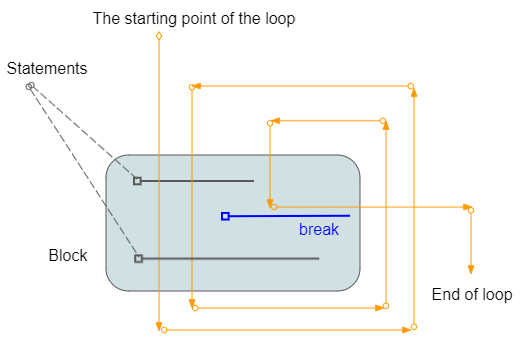Example:
loop_break_ex1.ts
``````
console.log("Break example");

let y = 2;
while (y < 15) {
console.log('----------------------');
console.log(`y = \${y}`);
// If y = 5 then exit the loop.
if (y == 5) {
break;
}
// Increase value of x by 1
y = y + 1;
console.log(`y after + 1 = \${y}`);
}
console.log("Done!");
``````
Output:
``````
Break example
----------------------
y = 2
y after + 1 = 3
----------------------
y = 3
y after + 1 = 4
----------------------
y = 4
y after + 1 = 5
----------------------
y = 5
Done!
``````

## 8- continue statement in the loop

continue is a statement that can appear in a loop. When the continue statement is encountered, the program will skip the lines below the continue and execute the next iteration (If the condition is still true).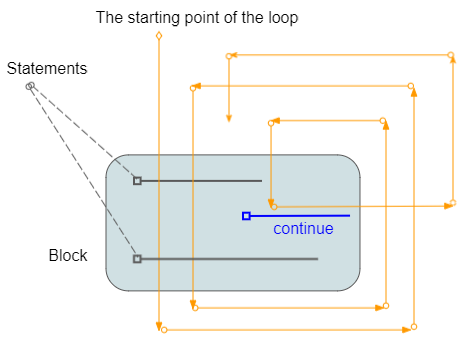Example:
``````
console.log('Continue example');
let z = 2
while (z < 7) {
console.log('----------------------')
console.log(`z = \${z}`);

if (z % 2 == 0) {
z = z + 1;
continue;
}
else {
z = z + 1;
console.log(`z after + 1 = \${z}`);
}
}
console.log('Done!');
``````
Output:
``````
Continue example
----------------------
z = 2
----------------------
z = 3
z after + 1 = 4
----------------------
z = 4
----------------------
z = 5
z after + 1 = 6
----------------------
z = 6
Done!
``````

## 9- Labeled Loops

TypeScript allows you to put a label for a loop. This is a way to name a loop and it comes in handy when you use multiple nested loops in a program.
• You can use the "break labelX" statement to break the loop labeled labelX.
• You can use the "continue labelX" statement to continue the loop labeled labelX.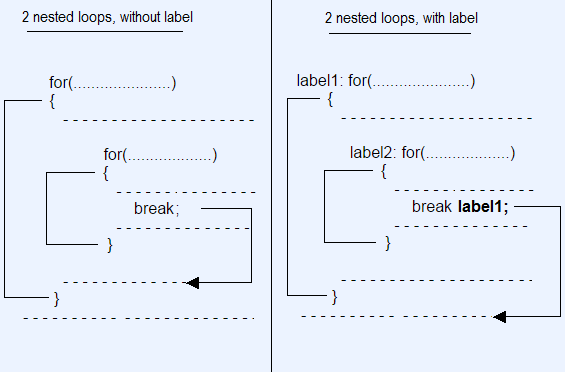Syntax:
``````
// for loop with Label.
label1: for( ... )  {

}
// while loop with Label.
label2: while ( ... )  {

}
// do-while loop with Label.
label3: do  {

} while ( ... );
``````
Example: Use labeled loops and break statement.
loop_break_labelled_ex1.ts
``````
console.log('Labelled Loop Break example');
let i = 0;
label1: while (i < 5) {
console.log('----------------------');
console.log(`i = \${i}`);
i++;
label2: for (let j = 0; j < 3; j++) {
console.log(`  --> \${j}`);
if (j > 0) {
// Exit the loop with label1.
break label1;
}
}
}
console.log('Done!');
``````
Output:
``````
Labelled Loop Break example
----------------------
i = 0
--> 0
--> 1
Done!
``````
Example: Use labeled loops and continue statement.
loop_continue_labelled_ex1.ts
``````
let j = 0;
label1: while (j < 5) {
console.log(`outer j= \${j}`);
j++;
label2: for (let k = 0; k < 3; k++) {
if (k > 0) {
continue label2;
}
if (j > 1) {
continue label1;
}
console.log(`inner j= \${j} , k= \${k}`);
}
}
``````
Output:
``````
outer j= 0
inner j= 1 , k= 0
outer j= 1
outer j= 2
outer j= 3
outer j= 4
``````

#### View more Tutorials:

Maybe you are interested

These are online courses outside the o7planning website that we introduced, which may include free or discounted courses.

•Super HTML5 Scratch. Learn All best codes Step by step
•Introduction To SQL Server Reporting Services -SSRS
•JavaFx Tutorial For Beginners
•Java for Intermediate Users
•React for Beginners: A Complete Guide to Getting Started
•Publish Games on Android, iTunes, and Google Play with UE4
•Python MySql From Scratch
•Filters in AngularJS
•The Web Development Course: HTML5, CSS3, JavaScript
•Java, JSP and JDBC programming guides for complete BEGINNER
•Beginner API development in Node, Express, ES6, & MongoDB
•Create Complete Web Applications easily with APEX 5
•Dart and Flutter: The Complete Developer's Guide
•Master Hibernate and JPA with Spring Boot in 100 Steps
•Oracle APEX Techniques
•Build an AirPods finder app in Swift - iOS 13 - Xcode 11
•What's New in C#7 and C# 8
•Create Dynamic web Forms with jQuery
•Flappy Bird Clone - The Complete SFML C++ Game Course
•iOS 13 - How to Make Amazing iPhone Apps: Xcode 11 & Swift 5
•Mastering Thymeleaf with Spring Boot
•SUPER Efficient with Flutter - Tips & Tricks for Intellij
•Oracle: The Complete SQL Guide (Certification: 1Z0-061/ 071)
•Create Ajax Chat App with PHP & Mysql
•Python 2000: Beyond The Basics